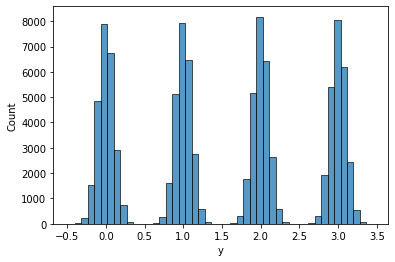# Effective Sample Size very low for Gaussian Mixture Model

I’m new to NumPyro, and I’m learning it with an aim to replace my current Stan model with Numpyro and achieve potential speedup.

I wrote a simple Gaussian Mixture Model - based on the model here, but with an added constraint that the cluster means be ordered. I’m testing it using a dataset of N = 100000 points, K = 4 clusters.

The sampler runs in ~20 minutes when using 2 chains and 1000 samples + 1000 warmups per chain, which is really fast! However, the effective sample size ess_bulk is only 3 for the means and std-devs of the clusters, which is extremely low.

I’ve created a notebook with my model, data and results - Gaussian Mixture Model - Google Colab

Also sharing here for convenience

Model

``````def gmm_model(y, K):
#mixture component probabilities
lambdas = numpyro.sample("lambdas", dist.Dirichlet(concentration=jnp.ones(K)))

#mu is vector of cluster means, should be an order vector drawn from a Normal distribution.
#Using a transformed distribution with an ordered transform.
mu = numpyro.sample("mu", dist.TransformedDistribution(dist.Normal(0, 10).expand([K]), transforms.OrderedTransform()))

#I'm guessing this is equivalent to expand()? Maybe it does stuff in parallel, but expand should do that too?
with numpyro.plate("K", K):
#sigma is vector of cluster stddevs
sigma = numpyro.sample("sigma", dist.HalfNormal(scale=0.1))

with numpyro.plate("N", len(y)):
#cluster_idx is vector of index to which cluster a data point belongs to.
#add infer={'enumerate': 'parallel'} to tell MCMC to marginalize discrete variable.
cluster_idx = numpyro.sample("cluster_idx", dist.Categorical(lambdas), infer={'enumerate': 'parallel'})

#likelihood - y ~ normal(mu[c], sigma[c])
numpyro.sample("y", dist.Normal(loc=mu[cluster_idx], scale=sigma[cluster_idx]), obs=y)
``````

Here’s what the data looks likeAnd here is the summary, which includes the effective sample size `ess_bulk`.

I’d appreciate any advice on how I can fix this problem: is my model misspecified, or do I just need to run with more samples?

The dataset is large so you might need to use double precision `numpyro.enable_x64()`. When things go wrong, you can simplify the problem to diagnose the issue:

• use smaller max_tree_depth (e.g. 5 or 6) to improve the speed
• use 1 chain rather than multiple chains
• reduce the dataset size
• simplify the complexity of the model (e.g. using constant `sigma`)

to @fehiepsi 's point 10^5 data points is a lot. the posterior probably has very high curvature and may have difficult geometry. if your idea was “let’s make the inference problem easier by using lots of data” that isn’t necessarily going to be the case here. probably the inference algorithm will find it easier to navigate a less curved posterior with e.g. 10^3 or 10^4 data points

Thanks @fehiepsi and @martinjankowiak . This turned out to not be a numpyro-specific problem. I found that when I tried to jointly learn the cluster params (means/stds) and the cluster assignment for each data point, the model struggled because the posterior was multimodal. This was the issue with Stan as well. So I decide the break the process into two stages - a training (learning the cluster means/stds) and prediction (learning the cluster assignments). That worked well, and it is ~40x faster than Stan for N=10^5 data points!